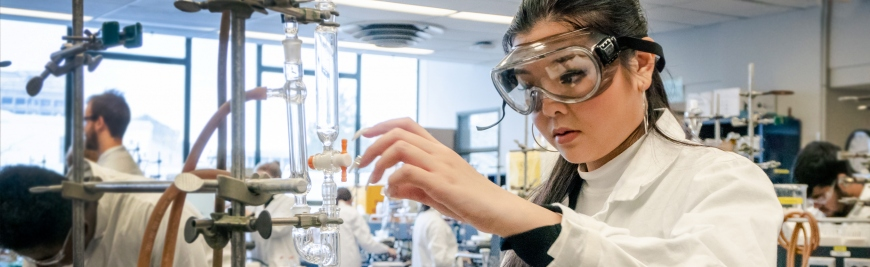## Research & Teaching Faculty# Influence of the 6th and 10th spatial harmonics on the peak shape of a quadrupole mass filter with round rods

 Title Influence of the 6th and 10th spatial harmonics on the peak shape of a quadrupole mass filter with round rods Publication Type Journal Article Year of Publication 2002 Authors Douglas, DJ, Konenkov, NV Journal Rapid Communications in Mass Spectrometry Volume 16 Pagination 1425-1431 Type of Article Article ISBN Number 0951-4198 Keywords 2ND, ION-TRAP, REGION, SIMULATION Abstract The influence of the ratio of the rod radius, r, to field radius, r(0), on the peak shape for a linear quadrupole mass filter constructed with round rods has been investigated. The expansion of the potential in multipoles, phi(N),Phi(x,y)= Sigma(N=0)(infinity)A(N)phi(N)/r(0)(N), has been considered, and the peak shape and resolution have been determined by numerical calculation of ion trajectories in quadrupoles with different ratios, r/r(0). Geometries that make the dodecapole term (A(6)) zero (r/(0) = 1.14511) do not give the best performance because the contribution of the 20-pole term, A(10), must be considered. The optimum ratio is r/r(0) approximate to 1.13. With this ratio the dodecapole term (A(6)) is ca. 1 x 10(-3), but its effects are partially compensated by the A(10) term which has similar magnitude, but opposite sign. Copyright (C) 2002 John Wiley Sons, Ltd. URL ://000177131400003